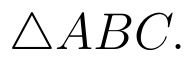Select Page

# Vector Algebra MCQ CBSE Maths 12 Science Solutions in English

Vector Algebra MCQ CBSE Maths 12 Science Solutions in English to enable students to get Solutions in a narrative video format for the specific question.

Expert Teacher provides Vector Algebra MCQ CBSE Maths 12 Science Solutions through Video Solutions in English language. This video solution will be useful for students to understand how to write an answer in exam in order to score more marks. This teacher uses a narrative style for a question from Vector Algebra not only to explain the proper method of answering question, but deriving right answer too.

Please find the question below and view the Solution in a narrative video format.

Question:

Solution Video in English:

You can select video Solutions from other languages also. Please check Solutions in ( Hindi )

## Similar Questions from CBSE, 12th Science, Maths, Vector Algebra

Question 1 : The two vectorsandrepresent the two sides AB and AC, respectively ofFind the length of the median

Question 2 : Find the scalar quantities :
(i)     Work                     (ii)      Force

Question 3 : Find |x|, if for a unit vector a,(x - a).(x + a) = 12. (View Answer Video)

Question 4 :  Find the sum of the vectorsand(View Answer Video)

Question 5 : Write the position vector of the point which divides the join of points with position vectorsandin the ratio 2:1.  (View Answer Video)

### Application of Integrals

Question 1 : Using integration, find the area of the triangle ABC, where A is (2,3), B is (4,7) and C is (6,2). (View Answer Video)

Question 2 : Using integration, find the area of theco-ordinates whose vertices are P(2,0), Q(4, 5) and R(6,3). (View Answer Video)

Question 3 : Find the area of the circlewhich is interior to the parabola(View Answer Video)

Question 4 : Find the area of the region. (View Answer Video)

Question 5 : Area lying in the first quadrant and bounded by the circleand the lines x =0 and x = 2 is (View Answer Video)

### Inverse Trigonometric Functions

Question 1 : Find the value ofWhat value do you learn from it ? (View Answer Video)

Question 2 : Write the value of(View Answer Video)

Question 3 : Write the principal value of(View Answer Video)

Question 4 : Ifthen find x. (View Answer Video)

Question 5 : Write the value of(View Answer Video)

### Matrices

Question 1 : If, find (x-y). (View Answer Video)

Question 2 : Compute:. (View Answer Video)

Question 3 :  Find the value of y, if(View Answer Video)

Question 4 : Compute:. (View Answer Video)

Question 5 : If A is a square matrix such that,then find the simplified value of:. (View Answer Video)